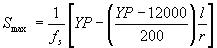Change List for this Rule

Original Version

Rule 48.2

48.2   Steel

The safety factors specified in Rule 44 shall be applied as follows to structural steel:

Tension and Bending: The yield point, 33,000 pounds per square inch, shall be divided by the safety factor to determine the maximum allowable working stress.

Compression: The maximum allowable working stress shall be calculated by the following formula:Where Smax = maximum allowable working stress, lbs per square inch

fs = safety factor specified in Rule 44

YP = yield point of the steel, 33,000 lbs. per sq. in.

l = unsupported length of member, inches

r = radius of gyration of member, inches

Shear: The ultimate tensile strength, 60,000 pounds per square inch, shall be multiplied by 2/3 and divided by the safety factor specified in Rule 44 to determine the maximum allowable working stress.

Where the figures given are used, structural steel shall conform to Standard Specifications A7�39 of American Society for Testing Materials for carbon steel of structural quality. Other values may be used for steel of other strength provided the yield point and ultimate tensile strength are determined by test.

Strikeout and Underline Version

Rule 48.2

48.2   Steel

The safety factors specified in Rule 44 shall be applied as follows to structural steel:

Tension and Bending: The yield point, 33,000 pounds per square inch, shall be divided by the safety factor to determine the maximum allowable working stress.

Compression: The maximum allowable working stress shall be calculated by the following formula:Where Smax = maximum allowable working stress, lbs per square inch

fs = safety factor specified in Rule 44

YP = yield point of the steel, 33,000 lbs. per sq. in.

l = unsupported length of member, inches

r = radius of gyration of member, inches

Shear: The ultimate tensile strength, 60,000 pounds per square inch, shall be multiplied by 2/3 and divided by the safety factor specified in Rule 44 to determine the maximum allowable working stress.

Where the figures given are used, structural steel shall conform to Standard Specifications A7�39 of American Society for Testing Materials for carbon steel of structural quality. Other values may be used for steel of other strength provided the yield point and ultimate tensile strength are determined by test.

Where American Society for Testing Materials (ASTM) A36-97 steel is used, the yield point shall be taken as 36,000 pounds per square inch (36ksi) and the tensile strength shall be taken as 58ksi.  If other grades of steel are used, the yield point and ultimate strength used to calculate maximum working stress shall correspond to the minimum values specified in the appropriate ASTM specification for the grade of steel used.

Final Version

Rule 48.2

48.2   Steel

The safety factors specified in Rule 44 shall be applied as follows to structural steel:

Tension and Bending: The yield point, 33,000 pounds per square inch, shall be divided by the safety factor to determine the maximum allowable working stress.

Compression: The maximum allowable working stress shall be calculated by the following formula:Where Smax = maximum allowable working stress, lbs per square inch

fs = safety factor specified in Rule 44

YP = yield point of the steel, 33,000 lbs. per sq. in.

l = unsupported length of member, inches

r = radius of gyration of member, inches

Shear: The ultimate tensile strength, 60,000 pounds per square inch, shall be multiplied by 2/3 and divided by the safety factor specified in Rule 44 to determine the maximum allowable working stress.

Where American Society for Testing Materials (ASTM) A36-97 steel is used, the yield point shall be taken as 36,000 pounds per square inch (36ksi) and the tensile strength shall be taken as 58ksi.  If other grades of steel are used, the yield point and ultimate strength used to calculate maximum working stress shall correspond to the minimum values specified in the appropriate ASTM specification for the grade of steel used.